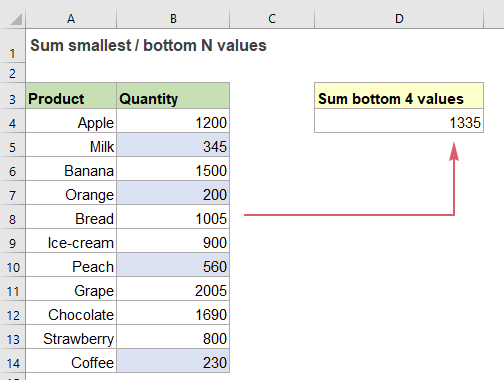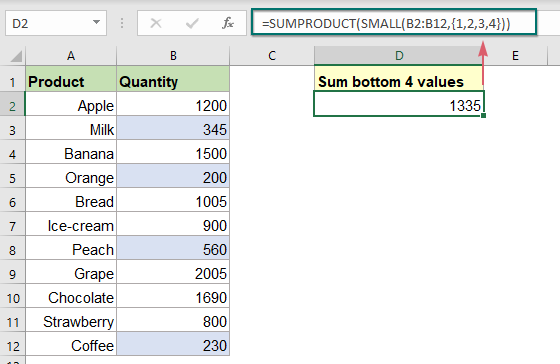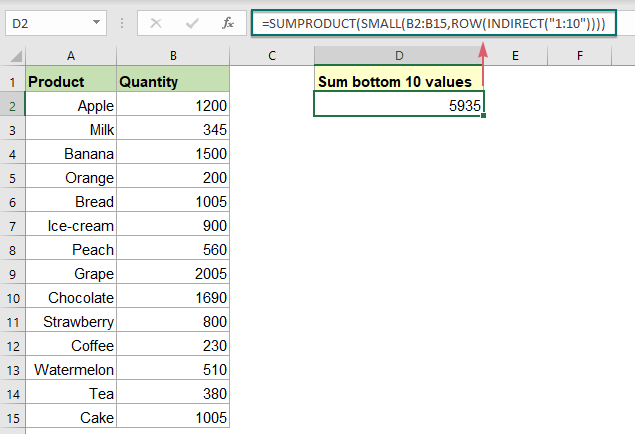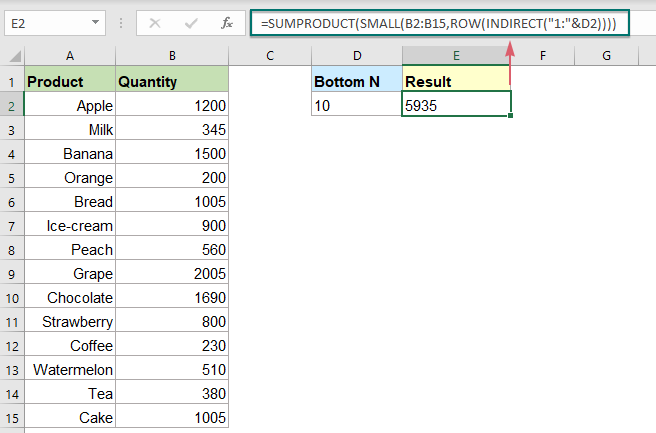Note: The other languages of the website are Google-translated. Back to English

## Sum smallest or bottom N values in Excel

In Excel, it is easy for us to sum a range of cells by using the SUM function. Sometimes, you may need to sum the smallest or bottom 3, 5 or n numbers in a data range as below screenshot shown. In this case, the SUMPRODUCT together with the SMALL function can help you to solve this problem in Excel.#### Sum smallest or bottom N values with SUMPRODUCT and SMALL functions

To sum the smallest n number in a data range, you can find the smallest n numbers by using the SMALL function, and then apply the SUMPRODUCT function to sum the array list directly, the generic syntax is:

=SUMPRODUCT(SMALL(range,{1,2,…,N}))
• range: The range of cells contains the numbers to be summed;
• N: Represents the Nth bottom value.

Here, I will sum the lowest 4 numbers in the range B2:B12, please enter or copy the below formula into a blank cell, and press Enter key to get the result:

=SUMPRODUCT(SMALL(B2:B12,{1,2,3,4}))##### Explanation of the formula:

=SUMPRODUCT(SMALL(B2:B12,{1,2,3,4}))

• SMALL(B2:B12,{1,2,3,4}):This SMALL function is used to return the 1st, 2nd, 3rd and 4th smallest values in the range B2:B12, you will get the array like this: {200,230,345,560}.
• SUMPRODUCT(SMALL(B2:B12,{1,2,3,4}))=SUMPRODUCT({200,230,345,560}): The SUMPRODUCT function sums all numbers in the array list to get the result:1335.

Tips: Solution when N becomes large:

Sometimes, you may have a large list of numbers, to sum the smallest 50 or more number in the range, it will be tedious to enter the number 1,2,3,4…50 into the SMALL function. So, here, you can use the ROW and INDIRECT functions for creating the array constant.

For example, to sum the lowest 10 values in a range, please apply the below formulas:

Manual input a number:

=SUMPRODUCT(SMALL(B2:B15,ROW(INDIRECT("1:10"))))Use a cell reference:

=SUMPRODUCT(SMALL(B2:B15,ROW(INDIRECT("1:"&D2))))#### Relative function used:

• SUMPRODUCT:
• The SUMPRODUCT function can be used to multiply two or more columns or arrays together, and then get the sum of products.
• SMALL:
• The Excel SMALL function returns a numeric value based on its position in a list when sorted by value in ascending order.

#### More articles:

• Sum Smallest Or Bottom N Values Based On Criteria
• In previous tutorial, we have discussed how to sum the smallest n values in a data range. In this article, we will perform a further advanced operation – to sum the lowest n values based on one or more criteria in Excel.
• Subtotal Invoice Amounts By Age In Excel
• To sum the invoice amounts based on age as below screenshot shown may be a common task in Excel, this tutorial will show how to subtotal invoice amounts by age with a normal SUMIF function.
• Sum All Number Cells Ignoring Errors
• When summing a range of numbers which contain some error values, the normal SUM function will not work correctly. To sum only numbers and skip the error values, the AGGREGATE function or the SUM along with the IFERROR functions can do you a favor.

### The Best Office Productivity Tools

#### Kutools for Excel - Helps You To Stand Out From Crowd

Would you like to complete your daily work quickly and perfectly? Kutools for Excel brings 300 powerful advanced features (Combine workbooks, sum by color, split cell contents, convert date, and so on...) and save 80% time for you.

• Designed for 1500 work scenarios, helps you solve 80% Excel problems.
• Reduce thousands of keyboard and mouse clicks every day, relieve your tired eyes and hands.
• Become an Excel expert in 3 minutes. No longer need to remember any painful formulas and VBA codes.
• 30-day unlimited free trial. 60-day money back guarantee. Free upgrade and support for 2 years.#### Office Tab - Enable Tabbed Reading and Editing in Microsoft Office (include Excel)

• One second to switch between dozens of open documents!
• Reduce hundreds of mouse clicks for you every day, say goodbye to mouse hand.
• Increases your productivity by 50% when viewing and editing multiple documents.
• Brings Efficient Tabs to Office (include Excel), Just Like Chrome, Firefox, And New Internet Explorer.